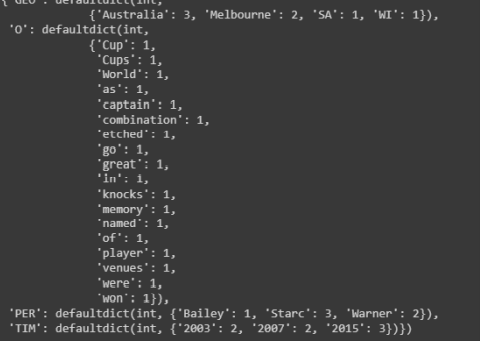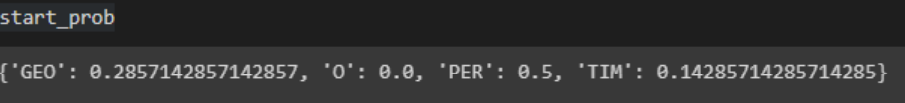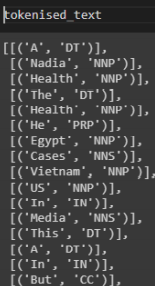Search

# Named-entity recognition model using the Hidden Markov Model

Problem Statement 1

You have been given a small dataset of sentences that are from a sports newspaper (HMM_Train_Sentences.txt), and you are also provided with the NER tagging of these sentences in a separate file (HMM_Train_NER.txt). The objective is to build a Named-entity recognition model using the Hidden Markov Model.

Dataset Description

Dataset: HMM_Train_Sentences.txt and HMM_Train_NER.txt HMM_Train_Sentences.txt contains few sentences from a newspaper and HMM_Train_NER.txt contains NER tags of words in those sentences

.ipynb file

Question 1.1

a. Read both the input data files and create two lists each for Words and NER tags.

b. Also, create the NER tags list for start and end token.

Hint: A start NER list should look like this with the starting tag of each sentence provided. Use the list index to obtain theseQuestion 1.2

a. Write functions for creating unigram, bigram tokens only for NER tags.

b. Also, calculate the NER Word count.

Hint: NER Word count is used to get how many times a word has occurred with respect to that NER. It should look like this.Question 1.3

a. Create HiddenMarkovModel (from pomegranate library) to identify entities for words.

b. Calculate the start, end, and emission probabilities.

Hint: Start probabilities and end probabilities should give probabilities of the tag that might occur at the start and end, respectively.To calculate emission probability, use this reference: First, calculate the emission and then the statesc. Calculate the transitional probability. Hint for calculation:Where NER_count_bg is the bigram count, and NER_count_ug is the unigram count.

d. Predict entities for this sentence, “Starc named 2015 Australia player.”

Problem Statement 2

You are provided with a corpus that has a lot of sentences with POS tagging completed (CRF_POS_dataset.csv). The objective is to use this dataset and build a Condition Random Field (CRF model) with sequence modeling

Dataset Description

Dataset: CRF_POS_dataset.csv

CRF_POS_dataset contains a lot of sentences. The dataset also contains POS tag of each word

.ipynb file 2

Question 2.1

a. Perform appropriate data cleaning & preprocessing steps

b. Then, tokenize the dataset.

Hint: Group the sentences by their serial number given. And then for each word, tag along with the POS. The output should be a list and ideally look like thisQuestion 2.2

a. Extract features from the text. Add features like whether the word is in lower case, is it a title or is it a digit and what is its POS tag, whether it is at the beginning of the sentence or at the end of the sentence. These features should be part of the X variable. And Y should be the target variable, i.e., POS of the particular word

Hint: Create a function to get these detailsThe output should look like below:

X Yb. Use sklearn_crfsuite and build a CRF model.

c. Also, run predictions on the same dataset.

Hint: Use CRF from crfsuite and build the model and get the predictions using cross_val_predict from sklearn.model_selection package. The prediction should ideally provide the output POS tags for words in the X dataset.Question 2.3

a. Build a flat classification report for each POS that is present in the corpus

and calculate the accuracy, F1 score

Hint: Use flat_classification_report from sklearn_crfsuite,metrics package to do this. The output should look like belowContact Us to get complete solution of above problem with an affordable price at realcode4you@gmail.com